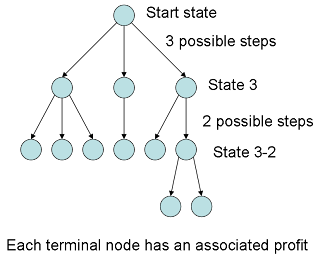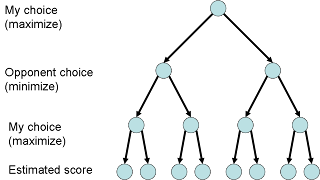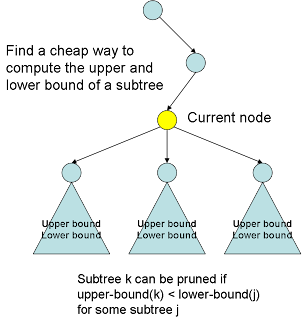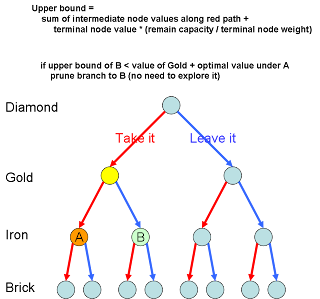## Saturday, July 5, 2008

### Branch and Bound Algorithm

Branch and Bound is a tree pruning technique for solving optimization problem using search technique.

Optimization problem is trying to find a path which maximize (or minimize) the profit. This solution can be represented as a state-space tree. Each branch represents all the possible next steps. The goal is to find a path which returns the maximum profit.Knapsack problem

We have n kinds of items, 1 through n. Each item j has a value pj and a weight wj. The maximum weight that we can carry in the bag is c. How should we decide which item to pick such that the sum of value is maximized (optimized) while the sum of weight is less than c (fulfill constraint).Minmax algorithm

2 players make moves in turn. Now is Player 1's turn, how should he choose his move in order to minimize his maximum lost when look ahead N steps.Brute force approach
One naive approach is to layout all the possible combination of steps and calculate the profit of each path. This is exponential complexity.

So a tree pruning mechanism is usually employed to reduce the exponential explosion. Bound and branch is such a mechanism. The basic idea is to have a cheap way to compute the upper bound and lower bound of the profit of a subtree. If a subtree is know to have its upper bound lower than the lower bound of another subtree, then this subtree can be pruned because its best result is worse than the worst result of that another subtree.Knapsack solutionMinimax solution (Alpha-Beta search)